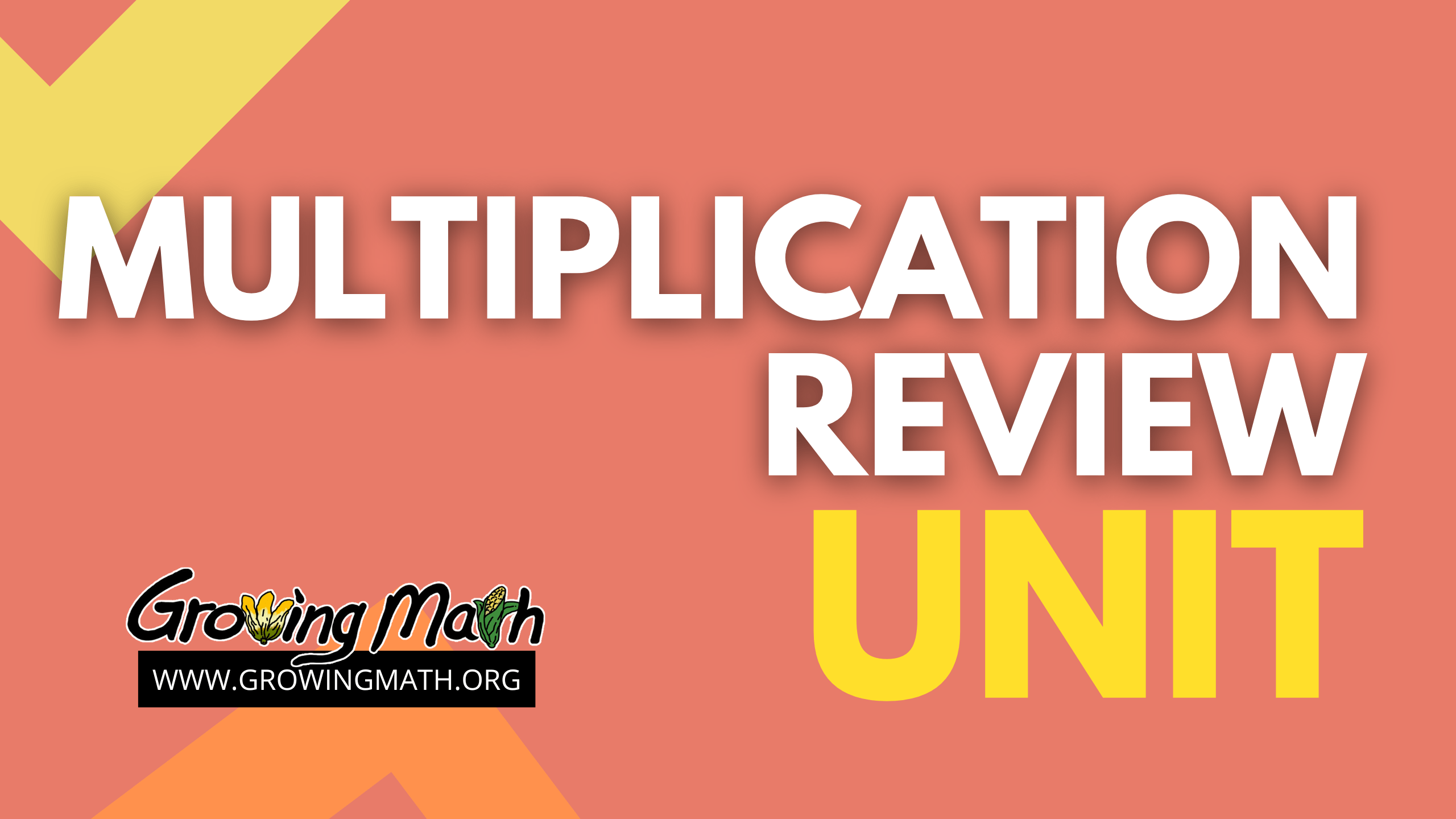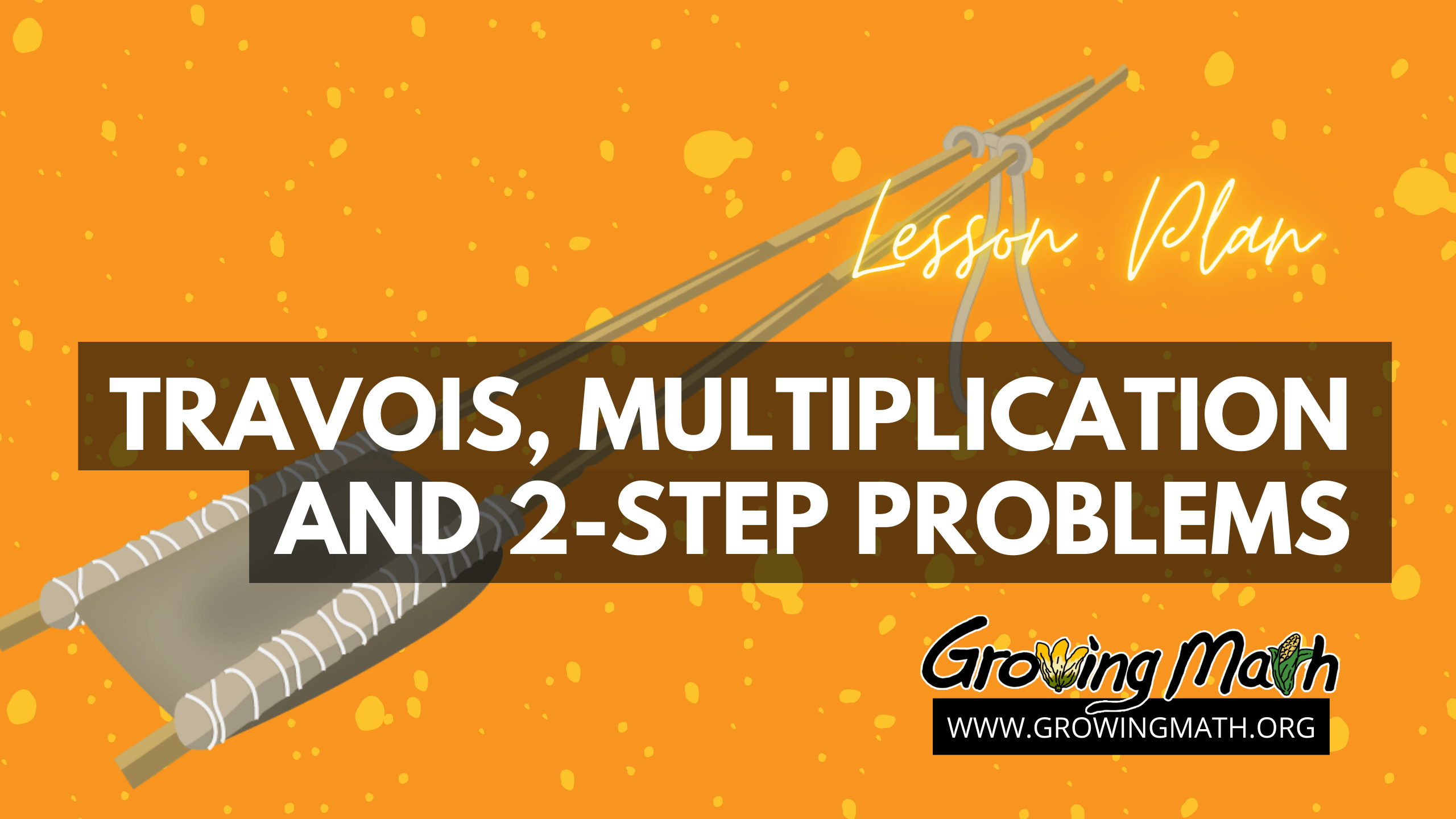# Category Archives: multiplication# Unit: Multiplication Review

## Standards

CCSS.MATH.CONTENT.3.OA.A.1 Interpret products of whole numbers, e.g., interpret 5 × 7 as the total number of objects in 5 groups of 7 objects each. For example, describe a context in which a total number of objects can be expressed as 5 × 7.
CCSS.MATH.CONTENT.3.OA.A.4 – Determine the unknown whole number in a multiplication or division equation relating three whole numbers.
CCSS.Math.Content.3.OA.B.5 Apply properties of operations as strategies to multiply and divide.
CCSS.MATH.CONTENT.3.OA.C.7– Fluently multiply and divide within 100

## Time

Lessons require from 10-40 minutes for a total of 90 minutes. These lessons are recommended more as a review or preview.

##Technology Required

Students need a device with web-browser – Chromebook, laptop or desktop computer, phone or tablet. If using a mobile device, we recommend downloading the Making Camp Premium app. It can also be played on the web on any computer.

## Unit Summary

Students who have recently learned multiplication tables still need frequent review. These five lessons present multiplication review in a variety of lengths and formats; games, cross-curricular formats integrating Ojibwe history, flash cards, virtual manipulatives, slide presentations and video.

## Ojibwe History Integrated with Math + History = Making Camp

That’s Making Camp in a nutshell, um, equation. Each of these only takes about 10 minutes, teaches Ojibwe (Native American) history , multiplication or division. You can do these at the beginning or end of class as a warm-up, as an assignment for those students who finish early or combine all three for a 30-minute lesson.

### Lesson 1: Making Camp – 10-minute multiplication review and Ojibwe history

Do this lesson first. Students sign in to the Making Camp game, play through the introduction, play a memory game and get a virtual dog.

### Lesson 2 – 10 Minute Mini-Lesson: Rabbit Stew & Multiplication

Students learn about what foods the Ojibwe people ate and how their diet changed when they were forced on to the reservation. They play a multiplication tic-tac-toe to snare rabbits and spend the points earned in the game to outfit their wigwam.

### Lesson 3 – Trade for a wigwam

Not strictly multiplication review but this is where students trade in the points they have earned in the prior two lessons for items for their wigwam.Students will watch two brief videos, one on building a wigwam and one on trading between tribes.Clicking on each item gives information on how that item was used by the Ojibwe

## Lesson 4 – Multiplication Review and Red River Carts

Students watch a video on the importance of the Red River cart in expanding trade. The teacher presents (or students may read) a presentation discussing Red River carts followed by two related word problems. The 20-minute lesson concludes with students playing Making Camp Premium, reinforcing multiplication facts and the Ojibwe history lesson learned.

## Lesson 5- Multiplication Terms

Students watch a video on multiplication terms then review terms with flash cards. Students quiz each other with flash cards. This 40-minute lesson closes with practicing multiplication and division by playing Making Camp Premium.# Single-Digit Multiplication Unit

## Standards

CCSS.MATH.CONTENT.3.OA.A.1 – Interpret products of whole numbers, e.g., interpret 5 × 7 as the total number of objects in 5 groups of 7 objects each.
CCSS.MATH.CONTENT.3.OA.A.3– Use multiplication and division within 100 to solve word problems in situations involving equal groups, arrays, and measurement quantities.
CCSS.MATH.CONTENT.3.OA.A.4– Determine the unknown whole number in a multiplication or division equation relating three whole numbers.
CCSS.MATH.CONTENT.3.OA.C.7– Fluently multiply and divide within 100, using strategies such as the relationship between multiplication and division (e.g., knowing that 8 × 5 = 40, one knows 40 ÷ 5 = 8) or properties of operations. By the end of Grade 3, know from memory all products of two one-digit numbers.

## Time

Each lesson will require 20-30 minutes. With the ten lessons, total time is approximately 5 hours spread over one to two weeks.

##Technology Required

Making Camp Premium plays in any browser and can also be downloaded on phones or tablets and played offline by students who have limited Internet access. The teacher will need a computer, for showing to students learning from home, and a projector if showing videos in the classroom. If the classroom does not have access to a projector, the videos can be skipped. Spirit Lake: The Game can be downloaded and played on Windows or Mac computers.

##Unit Summary

This 10-lesson unit teaches single-digit multiplication. Google slide presentations are provided within each lesson. Students are presented multiplication in a wide variety of formats, including, tables, number sentences, drawings, word problems and virtual manipulatives.

## Lessons 1-6: Multiplying one-digit numbers: 0 to 5

Students use visual drawings, manipulatives, and a number line to learn multiplication of one-digit numbers, coupled with their verbal explanations. Each lesson includes multiple assessments:

1. Students complete the multiplication tables – these can be shown with a projector (in class), on a screen, if teaching remotely, and on paper for students learning at home.
2. Students write their own number sentences using the multiplication learned each lesson.
3. Students complete the problems written by their classmates.
4. Optionally, students write word problems.
5. Optionally, student complete word problems written by their classmates.

At the end of lesson 5, students begin playing Making Camp Premium, which has teacher reports showing the number of multiplication problems attempted and answered correctly.

## Lessons 7-10: Multiplying one-digit numbers from 6 to 9

Students use visual drawings, manipulatives, and a number line to learn multiplication of one-digit numbers, coupled with their verbal explanations. Students play the Making Camp Premium game to reinforce learning. Those with access to a Mac or Windows computer can also play Spirit Lake. Each lesson will require 20-30 minutes.

• Students use visual drawings, manipulatives, and a number line to learn multiplication of one-digit numbers, coupled with their verbal explanations.
• Students will create number sentences independently and with a partner.
• Learning and memorizing multiplication patterns will improve later understanding of division.
• Determine the unknown whole number in a multiplication equation relating three whole numbers.
• Optional Brain Power activities have students write their own word problems.
• Students play games that reinforce memory and apply multiplication in word problems.# Travois, multiplication and 2-step problems

By Irish Pepito

## 📖 STANDARD

CCSS.MATH.CONTENT.3.OA.D.8 Solve two-step word problems using the four operations. Represent these problems using equations with a letter standing for the unknown quantity. Assess the reasonableness of answers using mental computation and estimation strategies including rounding.

30-45 minutes

## 📃 SUMMARY

In this lesson, the students will develop an understanding of the meanings of the four operations of whole numbers through activities and problems involving real life scenarios from Indigenous history. Students use properties of operations to calculate products of whole numbers, using increasingly sophisticated strategies based on these properties to solve using the four operations problems involving single-digit factors. It includes educational videos, games and video presentations that can be used for reviews and daily practice.

## 📲 TECHNOLOGY REQUIRED

Computer or mobile device

## 📚 LESSON

### Second, play a game

Let the students log in to Making Camp Premium to access the game. (Game links can be found under the GAMES tab.) The choices screen is shown above. Students are instructed to select NUMBERS from the screen above.

Students are tasked to play for 20 minutes. Students will demonstrate mastery in solving word problems like the one shown below and to be able to earn points within the game. For some students who aren’t able to master the standard will be given individualized instruction to master the concept.